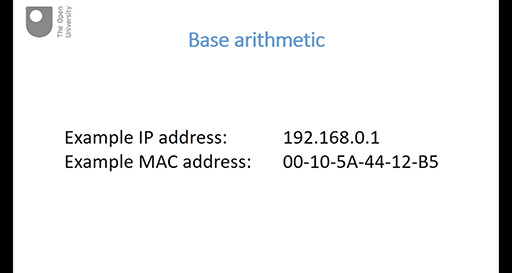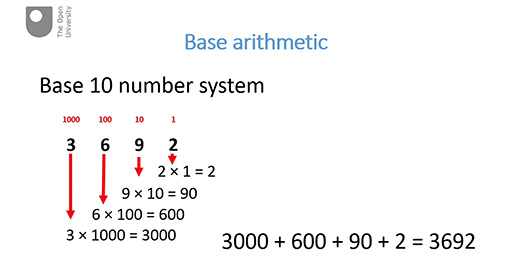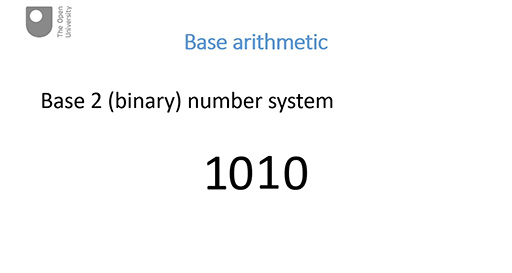Science, Maths & Technology

### Become an OU studentDiscovering computer networks: hands on in the Open Networking Lab

Start this free course now. Just create an account and sign in. Enrol and complete the course for a free statement of participation or digital badge if available.

# 7.1 Base arithmetic

In this part there is a slight side track from the focus on communications networks: instead you’ll take a short time to look at some arithmetic that will help you to understand the representation of network addresses. This is very fundamental arithmetic about how we count and how we represent numbers, and you may already be familiar with this. If this is the case, then you can treat this part as revision.

There are three videos in this part – each video is followed by an activity.

Watch the video below, which is about 3 minutes long.

## Base arithmeticInteractive feature not available in single page view (see it in standard view).

## Activity 1 Test yourself

5 minutes

• In the base 10 (decimal) number 242, the symbol 2 represents different values depending on its position. What are these values?

• The symbol ‘2’ on the left is in third place so a weighting of 100 is applied to it, giving it a value of 200. The symbol ‘2’ on the right is in first place so a weighting of 1 is applied to it giving it a value of 2.

• Working in the base 10 (decimal) number system, in a six-symbol number such as 495,362, what weighting is applied to the leftmost symbol? What does the symbol ‘4’ represent in the number 495,362?

• The leftmost symbol in our example is in sixth place so a weighting of 100,000 is applied to this symbol. So in the decimal number 495,362, the ‘4’ represents 400,000.

Watch the video below, which is about 2 minutes long.

## Exponential notationInteractive feature not available in single page view (see it in standard view).

## Activity 2 Test yourself

5 minutes

• 8 × 103 =

• 8 × 103 = 8000

• 4 × 105 =

• 4 × 105 = 400,000

• 7 × 107 =

• 7 × 107 = 70,000,000

Watch the video below, which is about 3 minutes long.

## Base 2 arithmeticInteractive feature not available in single page view (see it in standard view).

## Activity 3 Test yourself

5 minutes

Give the denary equivalents of the following binary numbers:

• 1110

• 1110 = (1 × 23) + (1 × 22) + (1 × 21) + (0 × 20) = 8 + 4 + 2 + 0 = 14

• 1001

• 1001 = (1 × 23) + (0 × 22) + (0 × 21) + (1 × 20) = 8 + 0 + 0 + 1 = 9

• 101101

• This is more difficult as you need to apply some higher binary weightings of 25 (16) and 26 (32).

So 101101 = (1 × 25) + (0 × 24) + (1 × 23) + (1 × 22) + (0 × 21) + (1 × 20) = 32 + 0 + 8 + 4 + 0 + 1 = 45.